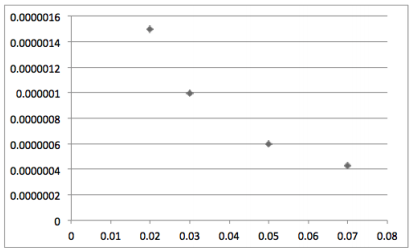# Question 1-Science Practice Test for the ACT

Mercury lamps emit ultraviolet radiation. What is the wavelength of these ultraviolet rays?

Note: On both attached graphs in “Read Text”, the x-axis should be labeled “wavelength” and the y-axis should be labeled “Hz”.

Energy travels through space by electromagnetic radiation (Table 1). There are many forms of radiant energy, such as sunlight, microwave oven energy, X-rays, and heat from a fireplace. All forms of electromagnetic radiation exhibit wavelike behaviour and travel at the speed of light (c) in a vacuum. Wavelength, frequenc and speed are the three primary characteristics of waves. Wavelength (λ) is the distance between two consecutive peaks in a wave. Frequency (ν) is the number of waves (cycles) per second that pass a given point in space. There is an inverse relationship between wavelength and frequency—short wavelength radiation has a high frequency, whereas long wavelength radiation has a low frequency.

Table 1: Classification of Electromagnetic Radiation

in Increasing Order
Gamma rays $$1 \cdot 10^{-12}$$
X-rays $$1 \cdot 10^{-10}$$
Ultraviolet rays $$1 \cdot 10^{-8}$$
Visible light spectrum $$4 \cdot 10^{-7} - 7 \cdot 10^{-7}$$
Infrared $$1 \cdot 10^{-4}$$
Microwaves $$1 \cdot 10^{-2}$$
-FM $$1$$
-Shortwave $$1 \cdot 10^2$$
-AM $$1 \cdot 10^4$$

Alfred and Gwen ran experiments to measure the wavelengths of various types of energy sources. Alfred used energy sources that emit microwave radiation, whereas Gwen used sources that emit infrared radiation. After determining the wavelengths of the energy sources used, they calculated the frequency of radiation for each source using the following formula for the speed of light:

c = λν, where
λ = wavelength [meters (m)]
ν = frequency [cycles per second = 1/s = Hertz (Hz)]
c = speed of light (m/s) = 2.9979 x 108 m/s

Frequency can be calculated by rearranging this equation to solve for frequency, ν = c/λ. Alfred and Gwen plotted frequency as a function of wavelength and the resulting graphs are shown in Figures 1 and 2.